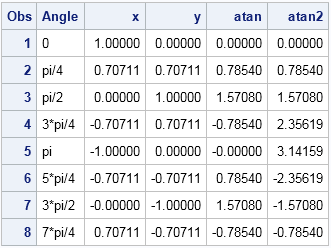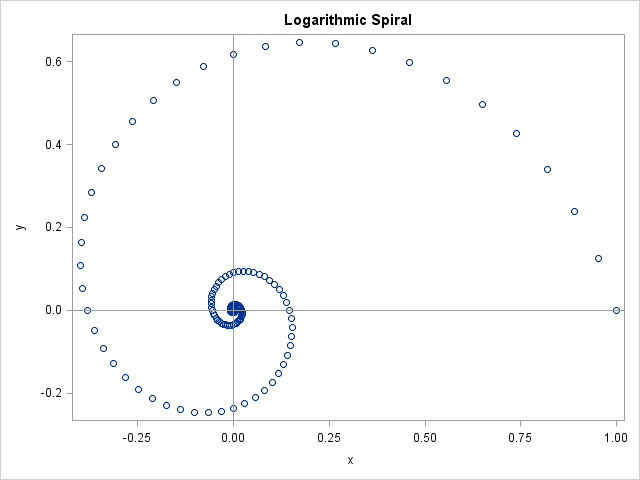Equations that involve trigonometric functions can have infinitely many solutions. For example, the solution to the equation tan(θ)=1 is θ = π/4 + kπ, where k is any integer. In order to obtain a unique solution to the equation, we define the "arc" functions: inverse trigonometric functions that return a principal value. For example, the Arctan function returns a principal value in the range (-π/2, π/2), such as Arctan(1) = π/4. In SAS software, the ATAN function computes the Arctan function.

You can extend the Arctan function in a natural way: given the coordinates (x, y) of a point in the plane, you can compute the angle formed between the x axis and the vector from the origin to (x, y). SAS provides the ATAN2 function for this computation. The ATAN2 function takes two arguments (the coordinates of an (x, y) pair) and returns the angle in the range (-π, π] that corresponds to the vector angle.

The ATAN and ATAN2 functions are supported in Base SAS, so you can call them from the DATA step or from the SAS/IML language. The following statements compute the points on the unit circle for several polar angles. The ATAN function evaluated at y/x returns the principal arctangent function. The ATAN2 function evaluated at (y, x) returns the polar angle in (-π, π]. Notice that order of the arguments for the ATAN2 function is the reverse of what you might expect!

```data Arctan; drop pi i theta; array a \$6 _temporary_ ("0" "pi/4" "pi/2" "3*pi/4" "pi" "5*pi/4" "3*pi/2" "7*pi/4") ; pi = constant("pi"); do i = 0 to 7; Angle = a[i+1]; theta = i*pi/4; /* angle in [0, 2*pi) */ x = cos(theta); /* (x,y) on unit circle */ y = sin(theta); atan = atan( y/x ); /* ATAN takes one argument */ atan2 = atan2(y, x); /* ATAN2 takes two arguments: Notice order! */ output; end; run; proc print; run;```Notice that the ATAN and ATAN2 functions agree for points that are in the first and fourth quadrants. Notice also that both functions return their values in radians. You can convert radians to degrees by multiplying the radian measure by 180/π.

### Compute angles along polar curvesSuppose that you have data points such as are shown in the scatter plot at the left. The ATAN2 function enables you to compute an angle in (-π, π] for a given data point. But to reconstruct the curve, you need to compute angles outside that interval. The scatter plot shows points that lie along a logarithmic spiral as computed by the following program.

```proc iml; pi = constant("pi"); /* create (x,y) coordinates of polar curve for (golden) logarithmic spiral r(theta) = a*exp(b*theta) */ a = 1; b = -0.3063; theta = do(0, 6*pi, pi/24)`; x = a*cos(theta)#exp(b*theta); y = a*sin(theta)#exp(b*theta); title "Logarithmic Spiral"; call scatter(x,y) other="refline 0 / axis=y; refline 0 / axis=x;";```

Recently I had data that looked like the scatter plot, and I needed to recover the angles that correspond to the points along the curve. If you naively use the ATAN2 function, all angles are translated into the interval (-π, π]. However, if the data are ordered according to the polar angle, as in these data, you can monitor the output of the ATAN2 function as you sequentially traverse the points along the curve. When one point is in the second quadrant but the next point is in the third quadrant, the ATAN2 function "jumps" by -2π across those two points. You can detect the discontinuity and add 2π to the value of the ATAN2 function evaluated all subsequent points along the curve. If you do this every time that the curve crosses the negative x axis, the resulting set of angles will correctly represent the polar angle along the curve.

This algorithm is implemented in the following SAS/IML module, which assumes that the polar angle increases along the curve. With slightly more complicated logic, you can derive the polar angle for points along any parametric curve in the plane.

```/* assume the polar angle is an increasing function along the curve */ start IncrAngle(x, y); theta = atan2(y,x); /* angle from center to point */ dif = dif(theta); /* difference of angles between adjacent pts */ idx = loc(dif^=. & dif<0); /* for which pts does angle decrease? */ n = nrow(dif); pi = constant("pi"); do i = 1 to ncol(idx); theta[idx[i]:n] = 2*pi + theta[idx[i]:n]; /* add 2*pi at discontinuities */ end; return(theta); finish;   phi = IncrAngle(x,y); /* test function for pts along logarithmic curve */```

The variable phi is a vector of increasing values. It is equal to the theta vector, which contained the original sequence of angles in [0, 6π].

### Summary

For any value, the ATAN function computes the principal arctangent of that value. For a point (x, y) in the plane, the ATAN2 function enables you to compute the angle formed between the x axis and the vector from the origin to (x, y). The ATAN2 function returns an angle in the range (-π, π], and the ATAN2 function has a jump discontinuity of 2π across the negative x axis. Using these facts, you can construct a continuous function that returns the polar angle for points along a parametric curve in the plane.

In my next blog post I will use this function to analyze data that seems to lie along a parametric curve in the plane.

ShareDistinguished Researcher in Computational Statistics

Rick Wicklin, PhD, is a distinguished researcher in computational statistics at SAS and is a principal developer of SAS/IML software. His areas of expertise include computational statistics, simulation, statistical graphics, and modern methods in statistical data analysis. Rick is author of the books Statistical Programming with SAS/IML Software and Simulating Data with SAS.

1.I have been wrestling with something similar. I have been trying to use SAS to calculate the angle formed by two flight segments, say Chicago to New York to Dallas. Have yet to be successful. Do you have an angle on the right direction?

•Yes. If u and v are two unit vectors, then the angle between them (theta) satisfies the equation u*v = cos(theta).
Therefore you can invert the equation to find theta. In the SAS/IML language I would use
proc iml;
u = {1 1};
v = {-1 -2};
w = u*v` / (norm(u)*norm(v));
theta = arcos( w );
deg = 180/constant("pi") * theta;
print theta deg;

•Thank you. This gives me a great place to start.

2.if i have starting point on 2D graph and an ending point, how can SAS calculat ethe angle between them, as if the starting point were the Origin?

•IF p=(x1, y1) and q=(x2, y2) then the dot product of those two vectors is computed as
p.q = |p| |q| cos(theta), where theta is the angle between them.
Therefore
pq = x1*x2 + y1*y2;
pNorm = sqrt(x1*x1 + y1*y1);
qNorm = sqrt(x2*x2 + y2*y2);
theta = arcos( pq / (pNorm*qNorm) );

In the SAS/IML language, the computation is easier since you can use vectors:

```proc iml; p = {2, 1}; q = {1, 3}; theta = arcos( p`*q / (norm(p)*norm(q)) ); /* angle in radians */ degree = theta*180/constant('pi'); /* angle in degrees */ print theta degree;```